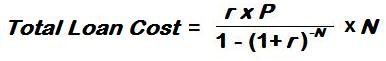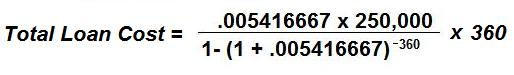CalcuNation

# Total Cost Mortgage Calculator

## Find the total cost over the life of a mortgage with this Total Cost Mortgage Calculator.

Principal Loan Amount:
Yearly Interest Rate: %
Total Months of the Loan:
A 30 year loan has 360 months, a 15 year loan has 180 months.

### How do you calculate the total cost of a mortgage?

Note: This calculator only shows the total accrued loan cost for the
principal and interest. This does not include other additional costs
associated with insurance or taxes.

To calculate the total cost for the life of a mortgage loan use the
formula:r = Monthly Interest Rate (in Decimal Form) =
(Yearly Interest Rate/100) / 12

P = Principal Amount on the Loan

N = Total # of Months for the loan ( Years on the loan x 12)

Example: The total cost for 30 year fixed rate loan, with a principal
of \$250,000, and a yearly interest rate of 6.5%:

r = (6.5 / 100) / 12 = .005416667

P = 250,000

N = (30 x 12) = 60The total cost for the life of the mortgage is \$568,861.22

### \$100 Promotion

Win \$100 towards teaching supplies! We want to see your websites and blogs.Enter Here

### Calculator Popups

Scientific Calculator
Simple Calculator

### Calculator Ideas

We use your calculator ideas to create new and useful online calculators.
Submit Calculator Idea